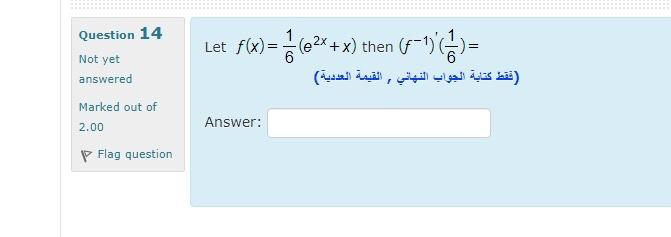### Create an Account

Home / Questions / Let f(x)= (62+ x) then (A-1)/() = (تقط كتابة الجواب النهائي , القيمة العددية) Question 14 ...

# Let f(x)= (62+ x) then (A-1)/() = (تقط كتابة الجواب النهائي , القيمة العددية) Question 14 Not yet answered Marked out of 2.00 P Flag question Answer:

Let f(x)= (62+ x) then (A-1)/() = (تقط كتابة الجواب النهائي , القيمة العددية) Question 14 Not yet answered Marked out of 2.00 P Flag question Answer:May 10 2021 View more View LessSubscribe To Get Solution